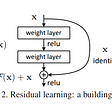# The Basic Classification of Thyroid Tumors on UltraSound Images using Deep Learning Methods

• 4c (Malign) • 5 (Malign)
`import osimport xml.etree.ElementTree as ETfrom natsort import natsortedimport pandas as pdfrom PIL import Imageimport numpy as npimport requestsfrom zipfile import ZipFilefrom io import BytesIOimport cv2import matplotlib.pyplot as pltimport tensorflow as tfimport mathimport randomfrom six.moves import xrangeimport collectionsimport string`
`def download_dataset(save_path):    r = requests.get("http://cimalab.intec.co/applications/thyroid/thyroid.zip")    print("Downloading...")    z = ZipFile(BytesIO(r.content))        z.extractall(save_path)    print("Completed...")# XML and Jpeg     def to_dataframe(path):    dirs=natsorted(os.listdir(path))    xml_list=[]    img_list=[]    for i in range(len(dirs)):        if '.xml' in dirs[i]:            xml_list.append(dirs[i])        if not '.xml'  in dirs[i]:            img_list.append(dirs[i])    xml_list=natsorted(xml_list)    img_list=natsorted(img_list)    tirads=[]    for j in range(len(xml_list)):        tree = ET.parse(path+'/'+xml_list[j])        a=tree.findall("./tirads")        if a[-1].text!=None:            case=[xml_list[j],a[-1].text]            tirads.append(case)    data=[]    for k in range(len(tirads)):        xml=tirads[k][:-4]        for z in range(len(img_list)):            if xml+'_1.jpg'==img_list[z] or xml+'_2.jpg'==img_list[z] or xml+'_3.jpg'==img_list[z]:                m=[img_list[z],tirads[k]]                data.append(m)    df = pd.DataFrame(data,columns =['Jpeg_Name', 'Tirads'])    return df`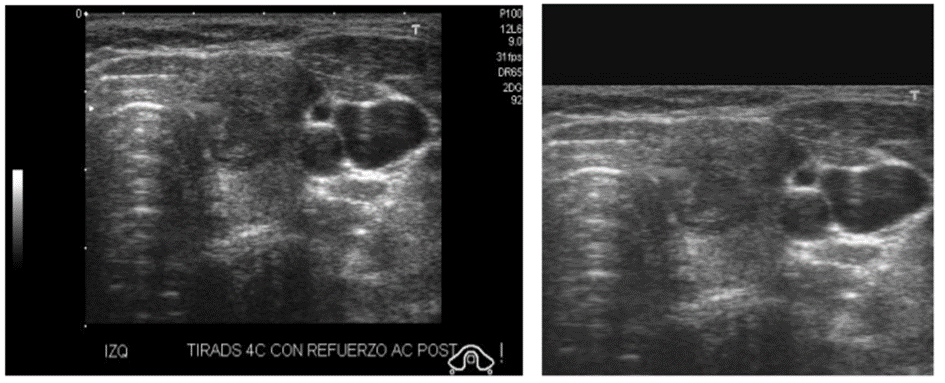Figure 1 — Original Image and Cropped and Resized Image which has given to model
`#Cropp Functiondef croping(img,x, y, w, h):    if abs(w)<abs(h):        img2=np.zeros([h,h])        img2[:,h-w:h]=img[y:y+h, x:x+w]    if abs(h)<abs(w):          img2=np.zeros([w,w])        img2[w-h:w,:]=img[y:y+h, x:x+w]    else:        return img    return img2def convert_one_channel(img):    #if some images have 3 channels , although they are grayscale image    if len(img.shape)>2:        img=img[:,:,0]        return img    else:        return img#Remove Fill area from Image and Resizeingdef crop_resize(path,resize_shape):    img=plt.imread(path)    img=convert_one_channel(np.asarray(img))        kernel =( np.ones((5,5), dtype=np.float32))    ret,thresh = cv2.threshold(img, 0, 255, cv2.THRESH_BINARY)    thresh = thresh.astype(np.uint8)    a1,b1=thresh.shape    thresh=cv2.morphologyEx(thresh, cv2.MORPH_OPEN, kernel,iterations=3 )    thresh=cv2.erode(thresh,kernel,iterations =5)    contours, hierarchy = cv2.findContours(thresh.copy(), cv2.RETR_TREE, cv2.CHAIN_APPROX_SIMPLE)    c_area=np.zeros([len(contours)])    for i in range(len(contours)):        c_area[i]= cv2.contourArea(contours[i])     cnts=contours[np.argmax(c_area)]        x, y, w, h = cv2.boundingRect(cnts)    roi = croping(img, x, y, w, h)    roi=cv2.resize(roi,(resize_shape),interpolation=cv2.INTER_LANCZOS4)    return roi# TO Data Matrixdef to_imgmatrix(resize_shape,path,df):    path=path+'/'      images=crop_resize(path+df["Jpeg_Name"],resize_shape)    for i in range (1,len(df["Jpeg_Name"])):        img=crop_resize(path+df["Jpeg_Name"][i],resize_shape)        images=np.concatenate((images,img))    images=np.reshape(images,(len(df["Jpeg_Name"]),resize_shape,resize_shape,1))    return imagesdef prepare_data(path,resize_shape):    df=to_dataframe(path)    data=to_imgmatrix(resize_shape,path,df)     return df,datadownload_dataset("/content/Data")#We want to resize 256x256 df,data=prepare_data("/content/Data",(256,256))`
`df.head()`
`# We need numeric categorydef to_categoricalmatrix(df):    #There are little categories, so i handled manually    Y=np.zeros([len(df["Tirads"])])    for i in range(len(df["Tirads"])):        if df["Tirads"][i]=="2":          Y[i]=0        if df["Tirads"][i]=="3":          Y[i]=1        if df["Tirads"][i]=="4a":          Y[i]=2        if df["Tirads"][i]=="4b":          Y[i]=3        if df["Tirads"][i]=="4c":          Y[i]=4        if df["Tirads"][i]=="5":          Y[i]=5    return Y# to integery=to_categoricalmatrix(df)y=tf.keras.utils.to_categorical(y, dtype='float32')`
`#normalize functiondef normalize(data):  for i in range(len(data)):    data[i,:,:,:]=data[i,:,:,:]*(1/np.max(data[i,:,:,:]))  return np.float32(data)  # we need noormalize to imagesx=normalize(data)`
`import randomrandom_number=random. randint(0,len(df["Tirads"]))plt.figure(figsize = (20,10))tit="Classification : "+np.str(df["Tirads"][random_number])plt.title(tit,fontsize = 40)plt.imshow(x[random_number,:,:,0],cmap="gray")`
`#Splitting test ,validation ,and trainx_train=np.copy(x[:300,:,:,:])x_test=np.copy(x[313:,:,:,:])x_valid=np.copy(x[300:313,:,:,:])y_train=np.copy(y[:300,:])y_valid=np.copy(y[300:313,:])y_test=np.copy(y[313:,:])`
`from tensorflow.keras import layers#Data Augmention for to prevent Overfitting and to improve accuracydata_augmentation1 = tf.keras.Sequential([ layers.experimental.preprocessing.RandomFlip(    "horizontal"),layers.experimental.preprocessing.RandomZoom(height_factor=(-0.2, 0.2),fill_mode="constant"),layers.experimental.preprocessing.RandomRotation(factor=(-0.2, 0.2),fill_mode="constant"),tf.keras.layers.experimental.preprocessing.RandomContrast(0.1)])x_train1=data_augmentation1(x_train)y_train1=np.copy(y_train)i=1#22while(i<22):  x_aug=data_augmentation1(x)  x_train1=np.concatenate((x_train1,x_aug),axis=0)  y_aug=np.copy(y)  y_train1=np.concatenate((y_train1,y_aug))  #20  if i == 20:    break  i += 1`
`def VGG19(input_shape,filters):    inputs=tf.keras.layers.Input(shape=input_shape)        x = tf.keras.layers.Conv2D(filters//16,(3,3), activation = 'relu', padding = 'same', kernel_initializer = 'he_normal')(inputs)    x=tf.keras.layers.Dropout(0.1)(x)    x = tf.keras.layers.Conv2D(filters//16,(3,3), activation = 'relu', padding = 'same', kernel_initializer = 'he_normal')(x)    x=tf.keras.layers.BatchNormalization()(x)    x = tf.keras.layers.MaxPooling2D(pool_size=(2, 2))(x)    x = tf.keras.layers.Conv2D(filters//8,(3,3), activation = 'relu', padding = 'same', kernel_initializer = 'he_normal')(x)    x=tf.keras.layers.Dropout(0.2)(x)    x = tf.keras.layers.Conv2D(filters//8,(3,3), activation = 'relu', padding = 'same', kernel_initializer = 'he_normal')(x)    x=tf.keras.layers.BatchNormalization()(x)        x = tf.keras.layers.MaxPooling2D(pool_size=(2, 2))(x)    x = tf.keras.layers.Conv2D(filters//4,(3,3), activation = 'relu', padding = 'same', kernel_initializer = 'he_normal')(x)    x=tf.keras.layers.Dropout(0.3)(x)    x = tf.keras.layers.Conv2D(filters//4,(3,3), activation = 'relu', padding = 'same', kernel_initializer = 'he_normal')(x)    x=tf.keras.layers.BatchNormalization()(x)    x = tf.keras.layers.Conv2D(filters//4,(3,3), activation = 'relu', padding = 'same', kernel_initializer = 'he_normal')(x)    x=tf.keras.layers.BatchNormalization()(x)    x = tf.keras.layers.MaxPooling2D(pool_size=(2, 2))(x)    x = tf.keras.layers.Conv2D(filters//2,(3,3), activation = 'relu', padding = 'same', kernel_initializer = 'he_normal')(x)    x=tf.keras.layers.Dropout(0.4)(x)    x = tf.keras.layers.Conv2D(filters//2,(3,3), activation = 'relu', padding = 'same', kernel_initializer = 'he_normal')(x)    x=tf.keras.layers.BatchNormalization()(x)    x = tf.keras.layers.Conv2D(filters//2,(3,3), activation = 'relu', padding = 'same', kernel_initializer = 'he_normal')(x)    x=tf.keras.layers.BatchNormalization()(x)    x = tf.keras.layers.MaxPooling2D(pool_size=(2, 2))(x)    x = tf.keras.layers.Conv2D(filters,(3,3),activation = 'relu', padding = 'same', kernel_initializer = 'he_normal')(x)    x=tf.keras.layers.Dropout(0.5)(x)    x = tf.keras.layers.Conv2D(filters,(3,3), activation = 'relu', padding = 'same', kernel_initializer = 'he_normal')(x)    x=tf.keras.layers.BatchNormalization()(x)    last = tf.keras.layers.Conv2D(filters,(3,3), activation = 'relu', padding = 'same', kernel_initializer = 'he_normal',name='top_conv')(x)        model=tf.keras.Model(inputs,last,name="VGG19")    return model`
`base_model=VGG19(input_shape=(256,256,1),filters=512)x = base_model.outputf=tf.keras.layers.Flatten(name="flatten")(x)#To prevent overfitting and unbalancing , used regularizerd2=tf.keras.layers.Dense(1024,activation="relu",kernel_regularizer=tf.keras.regularizers.l1_l2(0.00001))(f)dp9=tf.keras.layers.Dropout(0.5)(d2)d3=tf.keras.layers.Dense(1024,activation="relu")(f)dp10=tf.keras.layers.Dropout(0.5)(d2)final=tf.keras.layers.Dense(6,activation="softmax")(dp10)model = tf.keras.Model( inputs =[ base_model.input], outputs = final)`
`metrics=tf.keras.metrics.AUC(    num_thresholds=200, curve='ROC',    summation_method='interpolation')#categorical_crossentropymodel.compile(optimizer=tf.keras.optimizers.Adam(learning_rate=0.0001), loss="categorical_crossentropy",metrics=metrics)def lr_scheduler(epoch, lr):    decay_rate = 0.1    decay_step = 15    if epoch % decay_step == 0 and epoch:        return lr * decay_rate    return lr#after each 15 epochs , we want to decrease learning rate for converge to modellr_call = tf.keras.callbacks.LearningRateScheduler(lr_scheduler)epochs=35history=model.fit(x=[x_train1],y=[y_train1],batch_size=16,epochs=epochs,callbacks=[lr_call],validation_data=(x_valid,y_valid))`
`plt.figure(figsize = (20,10))plt.title('Loss')plt.plot(history.history['loss'], label='train')plt.plot(history.history['val_loss'], label='test')plt.legend()`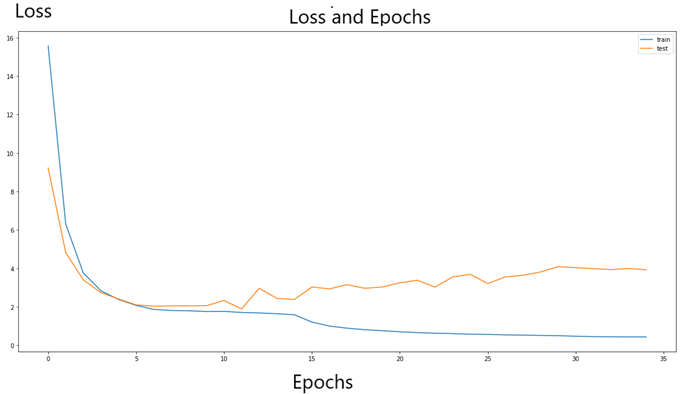Figure 3 — Loss and Epochs on Train and Validation Set
`import sklearnpredict=model.predict(x_test)auc = sklearn.metrics.roc_auc_score(y_test, predict)`
`y_test=np.reshape(y_test,(34*6))predict=np.reshape(predict,(34*6))from sklearn.metrics import roc_curvefrom sklearn.metrics import roc_auc_score# keep probabilities for the positive outcome onlyns_probs = [0 for _ in range(len(y_test))]# calculate scoresns_auc = roc_auc_score(y_test, ns_probs)lr_auc = roc_auc_score(y_test, predict)# summarize scoresprint('No Skill: ROC AUC=%.3f' % (ns_auc))print('Model: ROC AUC=%.3f' % (lr_auc))# calculate roc curvesns_fpr, ns_tpr, _ = roc_curve(y_test, ns_probs)lr_fpr, lr_tpr, _ = roc_curve(y_test, predict)# plot the roc curve for the modelplt.figure(figsize = (20,10))plt.title("ROC Curve",fontsize = 40)plt.plot(ns_fpr, ns_tpr,label='No Skill')plt.plot(lr_fpr, lr_tpr, label='Model')# axis labelsplt.xlabel('False Positive Rate')plt.ylabel('True Positive Rate')plt.rcParams["font.size"] = "15"# show the legendplt.legend()# show the plotplt.show()`
`#The GradCam observes the resultsdef make_gradcam_heatmap(img_array, model, last_conv_layer_name, classifier_layer_names ):    # First, we create a model that maps the input image to the activations    # of the last conv layer    last_conv_layer = model.get_layer(last_conv_layer_name)    last_conv_layer_model = keras.Model(model.inputs, last_conv_layer.output)    # Second, we create a model that maps the activations of the last conv    # layer to the final class predictions    classifier_input = keras.Input(shape=last_conv_layer.output.shape[1:])    x = classifier_input    for layer_name in classifier_layer_names:        x = model.get_layer(layer_name)(x)    classifier_model = keras.Model(classifier_input, x)    # Then, we compute the gradient of the top predicted class for our input image    # with respect to the activations of the last conv layer    with tf.GradientTape() as tape:        # Compute activations of the last conv layer and make the tape watch it        last_conv_layer_output = last_conv_layer_model(img_array)        tape.watch(last_conv_layer_output)        # Compute class predictions        preds = classifier_model(last_conv_layer_output)        top_pred_index = tf.argmax(preds)        top_class_channel = preds[:, top_pred_index]    # This is the gradient of the top predicted class with regard to    # the output feature map of the last conv layer    grads = tape.gradient(top_class_channel, last_conv_layer_output)    # This is a vector where each entry is the mean intensity of the gradient    # over a specific feature map channel    pooled_grads = tf.reduce_mean(grads, axis=(0, 1, 2))    # We multiply each channel in the feature map array    # by "how important this channel is" with regard to the top predicted class    last_conv_layer_output = last_conv_layer_output.numpy()    pooled_grads = pooled_grads.numpy()    for i in range(pooled_grads.shape[-1]):        last_conv_layer_output[:, :, i] *= pooled_grads[i]    # The channel-wise mean of the resulting feature map    # is our heatmap of class activation    heatmap = np.mean(last_conv_layer_output, axis=-1)    # For visualization purpose, we will also normalize the heatmap between 0 & 1    heatmap = np.maximum(heatmap, 0) / np.max(heatmap)    return heatmapfrom tensorflow import kerasimg_array=x_test[0,:,:,:]img_array=np.reshape(img_array,(1,256,256,1))preds = model.predict(img_array)last_conv_layer_name = "top_conv"classifier_layer_names = ["flatten"]                 # Generate class activation heatmapheatmap = make_gradcam_heatmap(    img_array, model, last_conv_layer_name, classifier_layer_names)img = keras.preprocessing.image.img_to_array(x_test[0,:,:,:])`
`from tensorflow import kerasimg_array=x_test[0,:,:,:]img_array=np.reshape(img_array,(1,256,256,1))preds = model.predict(img_array)last_conv_layer_name = "top_conv"classifier_layer_names = ["flatten"]                 # Generate class activation heatmapheatmap = make_gradcam_heatmap(    img_array, model, last_conv_layer_name, classifier_layer_names)img = keras.preprocessing.image.img_to_array(x_test[0,:,:,:])`

--

--

--

## More from S.Serdar Helli

AI Engineer ,MSc. Data Science, and Space Cowboy. https://serdarhelli.github.io

Love podcasts or audiobooks? Learn on the go with our new app.

## Functional programming for deep learning## 5 different ways to cross-validate your data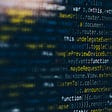## How fastai makes deep learning easy: classifying cancer cells using CNN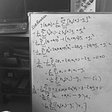## Understanding FastAI v2 Training with a Computer Vision Example- Part 1: The Resnet Model## Build your own Neural Network for CIFAR-10 using PyTorch## Machine Learning on Azure with automated predictions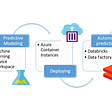## Deep Learning for 3D Point Clouds## Can Machines Think Using the Turing Test?## S.Serdar Helli

AI Engineer ,MSc. Data Science, and Space Cowboy. https://serdarhelli.github.io

## The DeepLab Family## More is not always good — Eye disease classification## Understanding Gradient Descent and Learning Rate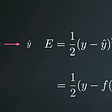## Residual Network: Code Explained